0
157

# SNAP Geometry Questions PDF [Most Important]

Geometry is an important topic in the Quant section of the SNAP Exam. Quant is a scoring section in SNAP, so it is advised to practice as much as questions from quant. This article provides some of the most important Geometry Questions for SNAP. One can also download this Free Geometry Questions for SNAP PDF with detailed answers by Cracku. These questions will help you practice and solve the Geometry questions in the SNAP exam. Utilize this PDF practice set, which is one of the best sources for practicing.

Question 1: In a triangle ABC, AB = AC = 8 cm. A circle drawn with BC as diameter passes through A. Another circle drawn with center at A passes through Band C. Then the area, in sq. cm, of the overlapping region between the two circles is

a) $16\pi$

b) $16(\pi – 1)$

c) $32(\pi – 1)$

d) $32\pi$

Solution: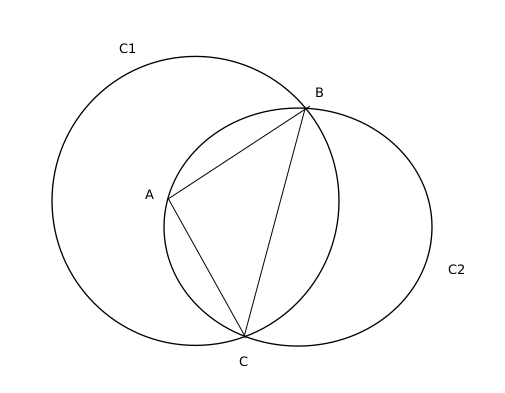BC is the diameter of circle C2 so we can say that $\angle BAC=90^{\circ\ }$ as angle in the semi circle is $90^{\circ\ }$

Therefore overlapping area = $\frac{1}{2}$(Area of circle C2) + Area of the minor sector made be BC in C1

AB= AC = 8 cm and as $\angle BAC=90^{\circ\ }$, so we can conclude that BC= $8\sqrt{2}$ cm

Radius of C2 = Half of length of BC = $4\sqrt{2}$ cm

Area of C2 = $\pi\left(4\sqrt{2}\right)^2=32\pi$ $cm^2$

A is the centre of C1 and C1 passes through B, so AB is the radius of C1 and is equal to 8 cm

Area of the minor sector made be BC in C1 = $\frac{1}{4}$(Area of circle C1) – Area of triangle ABC = $\frac{1}{4}\pi\left(8\right)^2-\left(\frac{1}{2}\times8\times8\right)=16\pi-32$ $cm^2$

Therefore,

Overlapping area between the two circles= $\frac{1}{2}$(Area of circle C2) + Area of the minor sector made be BC in C1

= $\frac{1}{2}\left(32\pi\right)\ +\left(16\pi-32\right)=32\left(\pi-1\right)$ $cm^2$

Question 2: The lengths of all four sides of a quadrilateral are integer valued. If three of its sides are of length 1 cm, 2 cm and 4 cm, then the total number of possible lengths of the fourth side is

a) 3

b) 4

c) 6

d) 5

Solution:

Sum of the three sides of a quadrilateral is greater than the fourth side.

Therefore, let the fourth side be

1+2+4>d or d<7

1+2+d>4 or d>1

Possible values of d are 2, 3, 4, 5 and 6.

Question 3: Suppose the medians BD and CE of a triangle ABC intersect at a point O. If area of triangle ABC is 108 sq. cm., then, the area of the triangle EOD, in sq. cm., is

Solution: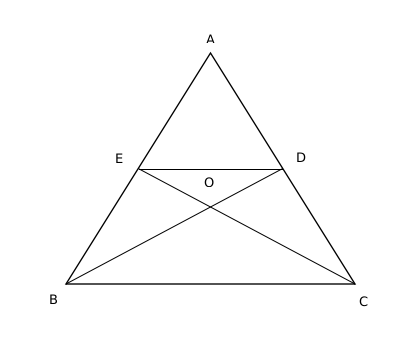Area of ABD : Area of BDC = 1:1

Therefore, area of ABD = 54

Area of ADE : Area of EDB = 1:1

Therefore, area of ADE = 27

O is the centroid and it divides the medians in the ratio of 2:1

Area of BEO : Area of EOD = 2:1

Area of EOD = 9

Question 4: The length of each side of an equilateral triangle ABC is 3 cm. Let D be a point on BC such that the area of triangle ADC is half the area of triangle ABD. Then the length of AD, in cm, is

a) $\sqrt{8}$

b) $\sqrt{6}$

c) $\sqrt{7}$

d) $\sqrt{5}$

Solution: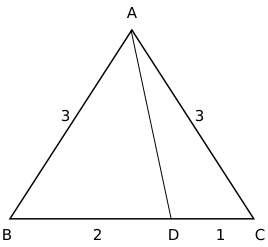Area of triangle ABD is twice the area of triangle ACD

$\angle\ ADB=\theta\$

$\frac{1}{2}\left(AD\right)\left(BD\right)\sin\theta\ =2\left(\frac{1}{2}\left(AD\left(CD\right)\sin\left(180-\theta\ \right)\right)\right)$

$BD\ =2CD$

Therefore, BD = 2 and CD = 1

$\angle\ ABC=\angle\ ACB=60^{\circ\ }$

Applying cosine rule in triangle ADC, we get

$\cos\angle\ ACD=\ \frac{\ AC^2+CD^2-AD^2}{2\left(AC\right)\left(CD\right)}$

$\frac{1}{2}=\ \frac{\ 9+1-AD^2}{6}$

$AD^2=7$

$AD=\sqrt{\ 7}$

Question 5: Regular polygons A and B have number of sides in the ratio 1 : 2 and interior angles in the ratio 3 : 4. Then the number of sides of B equals

Solution:

Let the number of sides of polygons A and B be n and 2n, respectively.

$\ \dfrac{\ \ \dfrac{\ \left(n-2\right)180}{n}}{\ \dfrac{\ \left(2n-2\right)180}{2n}}=\dfrac{3}{4}$

$\ \dfrac{\ \ \ n-2}{\ \ n-1}=\dfrac{3}{4}$

$\ \ \ \ 4n-8=3n-3$

$n=5$

The number of sides of polygon B is 2*5, i.e. 10.

Question 6: In triangle ABC, altitudes AD and BE are drawn to the corresponding bases. If $\angle BAC = 45^{\circ}$ and $\angle ABC=\theta\$, then $\frac{AD}{BE}$ equals

a) $\sqrt{2} \cos \theta$

b) $\frac{(\sin \theta + \cos \theta)}{\sqrt{2}}$

c) 1

d) $\sqrt{2} \sin \theta$

Solution: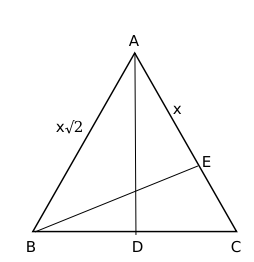It is given, Angle BAE = 45 degrees

This implies AE = BE

Let AE = BE = x

In right-angled triangle ABD, it is given $\angle ABC=\theta\$

$\sin\theta=\frac{AD}{AB}\$

$\sin\theta=\frac{AD}{x\sqrt{\ 2}}\$

$\sqrt{\ 2}\sin\theta=\frac{AD}{BE}\$

Question 7: Let ABCD be a parallelogram such that the coordinates of its three vertices A, B, C are (1, 1), (3, 4) and (−2, 8), respectively. Then, the coordinates of the vertex D are

a) (0, 11)

b) (4, 5)

c) (−3, 4)

d) (−4, 5)

Solution:

In a parallelogram, two diagonals of parallelogram bisects each other, which concludes that mid-point of both diagonals are the same.

Midpoint of AC = $\left(\ \frac{\ 1-2}{2},\ \frac{\ 1+8}{2}\right)$

Let the coordinates of vertex D be (x,y)

$\left(\ \frac{\ x+3}{2},\ \frac{\ y+4}{2}\right)=\left(\ \frac{\ 1-2}{2},\ \frac{\ 1+8}{2}\right)$

x = -4 and y = 5

Question 8: All the vertices of a rectangle lie on a circle of radius R. If the perimeter of the rectangle is P, then the area of the rectangle is

a) $\frac{P^2}{16} – R^2$

b) $\frac{P^2}{8} – 2R^2$

c) $\frac{P^2}{2} – 2PR$

d) $\frac{P^2}{8} – \frac{R^2}{2}$

Solution: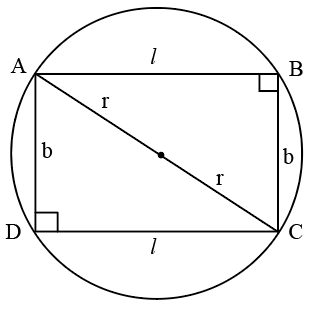$A=lb$

$l^2+b^2=4r^2$

$P\ =2\left(l+b\right)$

$\frac{P}{2}=l+b$

Squaring on both the sides, we get

$\frac{P^2}{4}=l^2+b^2+2lb$

$\frac{P^2}{4}=4r^2+2lb$

$\frac{P^2}{8}-2r^2=lb$

Question 9: A trapezium $ABCD$ has side $AD$ parallel to $BC, \angle BAD = 90^\circ, BC = 3$ cm and $AD= 8$ cm. If the perimeter of this trapezium is 36 cm, then its area, in sq. cm, is

Solution: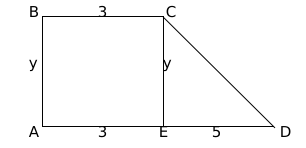CD = $\sqrt{\ y^2+25}$

$11+y+\sqrt{y^2+25}=36$

$\sqrt{y^2+25}=25-y$

$y^2+25=25^2+y^2-50y$

2y = 24

y = 12

Area of trapezium = $3y+\frac{5y}{2}=\frac{11y}{2}=\frac{11}{2}\left(12\right)=66$

Question 10: If a 30 meter ladder is placed against a wall such that it just reaches the top of the wall, if the horizontal distance between the wall and the base of the ladder is 1/3rd of the length of ladder, then the height of wall is :

a) 25 meter

b) $20\sqrt{2}$ meter

c) $20\sqrt{3}$ meter

d) 20 meter

Solution: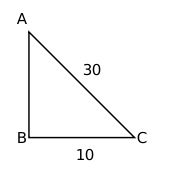Given AC = 30 m and BC = $\frac{1}{3}AC$ = $\frac{1}{3}\left(30\right)$ = 10 m

Applying pythagoras theorem,

$AB^2+\ BC^2=\ AC^2$

$AB^2$ = $30^2-10^2$ = 800

AB = $20\sqrt{\ 2}$

Question 11: A copper wire having length of 243m and diameter 4 mm was melted to form a sphere. Find the diameter of the sphere :

a) 17 m

b) 18 cm

c) 15 cm

d) 20 cm

Solution:

Volume of copper wire melted will be equal to volume of sphere.

Volume of copper wire = Area $\times\$ length = $\pi\ \left(\frac{2}{10}\right)^2\times\ 24300\ cm^3$

Let the radius of sphere be r

$\frac{4}{3}\pi\ r^{3\ \ }=\ \pi\ \left(\frac{2}{10}\right)^2\times\ 24300\ cm^3$

$r^3$ = 729

r = 9 cm

Diameter of sphere = 2(9) = 18 cm

Question 12: If a cuboidal box has height, length and width as 20 cm, 15 cm and 10 cm respectively. Then its total surface area is__________.

a) 1300$cm^{2}$

b) 1400$cm^{2}$

c) 1200$cm^{2}$

d) 1100$cm^{2}$

Solution:

Total surface area = 2(lb + bh + hl) = 2(150 + 200 + 300) = 1300 $cm^2$

Question 13: Angle bisectors of a parallelogram form a _____.

a) parallelogram

b) square

c) rhombus

d) rectangle

Solution: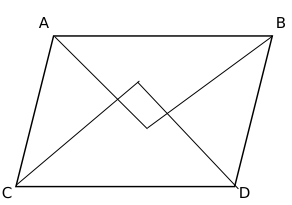Angular bisectors from A and B intersect at P, B and D intersect at Q, C and D intersect at R, and A and C intersect at S.
In a parallelogram ABCD, AC is parallel to BD.
$\angle\ CAB\ +\angle\ ACD\ =\ 180^{\circ\ }$
$\angle\ CAS\ +\angle\ ACS\ =\ 90^{\circ\ }$
In triangle CAS,
$\angle\ ASC\ =\ 180^{\circ\ }-90^{\circ\ }=90^{\circ\ }$
As all the angles of PQRS are $90^{\circ\ }$, PQRS is a rectangle.

Question 14: A tree bends due to heavy storm and now its peak touches the ground making an angle of $30^{\circ}$ with it. If the bent part is 20 metre long, find the original height of the tree in metres.

a) 10

b) 20/√3

c) 40

d) 30

Solution: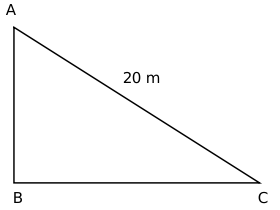BC is ground and it is given that $\angle\ ACB\ =30^{\circ\ }$
Actual length of the tree = AB + AC
$\sin30^{\circ\ }=\frac{AB}{AC}$
AB = 20/2 = 10 cm
Length of the tree = AB + AC = 10 + 20 = 30 cm

Question 15: $ABC$ is a triangle, $\angle B=60^\circ, \angle C=45^\circ$, BC is produced to / extended till D so that $\angle ADB=30^\circ$, then given $\sqrt{3}=\frac{19}{11}$ and $BC \times CD=3^{4}\times 2^{3}\times\frac{11}{19}$, what will be the square of the altitude from A to BC?

a) 144

b) 324

c) 484

d) 1254

Solution: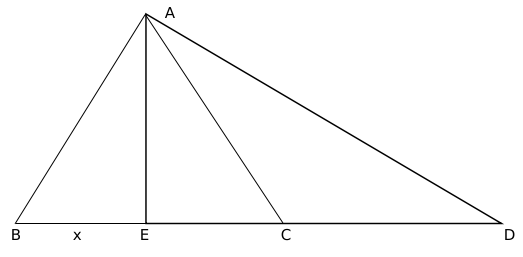AE is altitude from A on BC. $\angle\ AEC\ =\ 90^{\circ\ }$

It is given,

$\angle\ ABC\ =\ 60^{\circ\ },\ \angle\ ACB\ =\ 45^{\circ\ }$

In triangle ABE,

AE = $\sqrt{\ 3}x$

As $\angle\ EAC\ =\angle\ ECA$, EC = $\sqrt{\ 3}x$

In triangle AED, $\angle\ EAD\ =\ 60^{\circ\ }$

Therefore, CD = $3x-\sqrt{\ 3}x$

It is given,

$BC\ \cdot\ CD\ =\ \frac{3^4.2^3.11}{19}$

$x\left(\sqrt{\ 3}+1\right)\sqrt{\ 3}\left(\sqrt{\ 3}-1\right)x\ =\ \frac{3^4.2^3.11}{19}$

$x\ =\ \frac{3^2.2.11}{19}$

AE = 9*2 = 18

$AE^2$ = 324

Question 16: ABCD is a rectangle in the clockwise direction. The coordinates of A are (1,3) and the coordinates of C are (5,1), the coordinates of vertices B and D satisfy the line $y=2x+c$, then what will be the coordinates of the mid-point of BC.

a) $(\frac{5}{2},\frac{7}{2})$

b) $(\frac{9}{2},\frac{5}{2})$

c) $(\frac{9}{5},\frac{7}{2})$

d) $(3,2)$

Solution: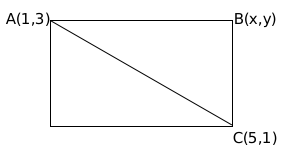Midpoint of AC passes through the line y = 2x + c

Midpoint of AC = (3,2)

2 = 6 + c

c = -4

Line passing through B is y = 2x – 4

(slope of AB)(slope of BC) = -1

$\left(\ \frac{\ y-1}{x-5}\right)\left(\ \frac{\ y-3}{x-1}\right)=-1$

Substituting y = 2x – 4 and solving, we get

B(2,0) or B(4,4)

B cannot lie on X-axis. Therefore, co-ordinates of B = (4,4)

Midpoint of BC = $\left(\frac{9}{2},\frac{5}{2}\right)$

Question 17: In a figure, $\triangle ABC$ is a right angled triangle at $C$, semicircles are drawn on $AC$, $BC$ and $AB$’s diameter. Find the area of the shaded region.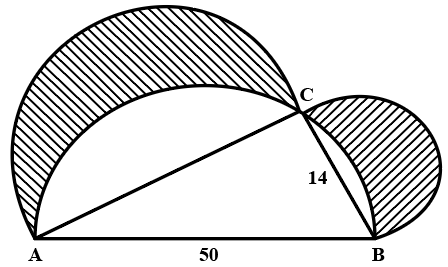Note: Figure not as per scale

a) 336

b) 676

c) 196

d) 556

Solution:

Area of shaded region = area of semicircle on BC + area of semicircle of AC – area of unshaded semicircle regions
Area of unshaded semicircle regions = area of semicircle on AB – area of triangle ABC
Given,
AB = 50, BC = 14
In triangle ABC, $AC^2+BC^2 = AB^2$
Solving we get,
AC = 48
Area of unshaded semicircle regions = $\pi\ \left(25\right)^2\$ – $\frac{1}{2}\times\ 48\times\ 14$ = $625\pi\ -336$
Area of shaded region = $576\pi\ +49\pi\ -625\pi\ +336$ = 336

Question 18: In the figure (not drawn to scale) given below, if $AD=CD=BC$ and angle $BCE = 81^\circ$, how much is the value of angle $DBC$?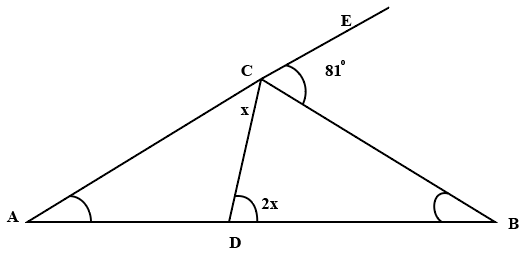a) $27^\circ$

b) $33^\circ$

c) $54^\circ$

d) $66^\circ$

Solution:

Given,
AD = DC, this implies angle ACD = angle DAC = x
DC = BC, this implies angle CDB = angle CBD = 2x
$\angle\ DCB$ = 180 – x – 81 = 99 – x
In triangle BCD, sum of the angles is 180 degrees
99 – x + 2x + 2x = 180
3x = 81
x = 27
$\angle\ DBC$ = 2x = 54 degrees

Question 19: A triangular park named ABC, is required to be protected by green fencing. The length of the side BC is 293. If the length of side AB is a perfect square, the length of the side AC is a power of two (2), and the length of side AC is twice the length of side AB. Determine how much fencing is required to cover the triangular park.

a) 1079

b) 1024

c) 1096

d) 1061

Solution:

ABC is a triangle and BC =  293
Let the length of AB = $x^2$
The length of AC = $2^y$
AC = 2AB
$2^y = 2.x^2$
$2^y-1 = x^2$
$2^y-1$ is a perfect square when y is odd.
Sum of any two sides of a triangle should be larger than the third side. Therefore,
$2^y + x^2$ > 293, 293 + $2^y$ > $x^2$ and 293 + $x^2$ > $2^y$
y > 8
If y = 9, AC = 512, x = 16; satisfies above equations.
If y = 11, AC = 2048, x = 32; doesn’t satisfy above equations.
Therefore, AB = 256 and AC = 512
Fencing required = 256 + 512 + 293 = 1061

Question 20: The angle of elevation of a glider from the deck of ship 25 meters above the sea surface is $30^\circ$ nd the angle of depression of the reflection of glider in the sea is $75^\circ$. Find the approximate height of the glider from the sea surface. $\sqrt{3}=\frac{19}{11}$

a) 46

b) 34

c) 29

d) 52

Solution: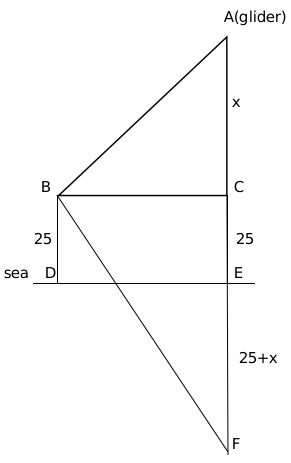It is given, angle ABC = 30 degrees and angle FBC = 75 degrees

$\tan\ 30^{\circ\ }=\frac{x}{BC}$

$BC\ =\ x\sqrt{\ 3}$

$\tan75^{\circ\ }=\ \frac{\ 50+x}{x\sqrt{\ 3}}$

$2+\sqrt{\ 3}\ =\ \frac{\ 50+x}{x\sqrt{\ 3}}$

Solving we get x = 9.1

Height of glinder from sea surface = 25+x $\approx\$ 34